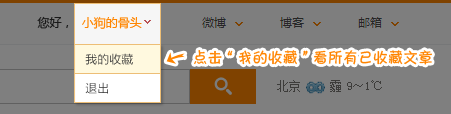# [500彩票网]大乐透第15027期：前区胆码01、12

2015年03月10日13:30   收藏本文

锦绣前程

龙头推荐：

01 12 15 首选1路，看淡2路。

凤尾推荐：

32 34 35 首选2路，看淡0路。

胆码推荐：

金胆：01

银胆：12

铜胆：35

胆码组：01+12+32+35

双胆：01+05、12+15、15+18、22+25、32+35

冷号推荐：18+25+35

重号推荐：15+22

尾号推荐：2+5+8

连号推荐：24+25、25+26、28+29、31+32、34+35

后起之秀

独 胆：01

单 注：01+07

三码小复式：01+05+07

四码小复式：01+06+05+07

五码小复式：01+05+06+07+09

号码组合：

非常5+5：

前区：01+12+24+32+35

后区：01+05+06+07+09

8码小复式：

前区：01+12+15+24+25+28+32+35

后区：01+05+07

12码大复式：

前区：01+05+12+15+18+22+24+25+26+28+32+35

后区：01+07

16码选号大底：

01+05+09+12+15+18+22+24+25+26+28+29+31+32+34+35(500彩票网)